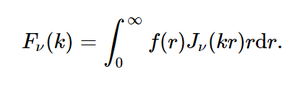# sympy.integrals.transforms.hankel_transform() in python

• Last Updated : 10 Jul, 2020

With the help of hankel_transform() method, we can compute the hankel transformation and returns the transformed function by using this method.hankel transformation

Syntax : hankel_transform(f, r, k, nu, **hints)

Attention geek! Strengthen your foundations with the Python Programming Foundation Course and learn the basics.

To begin with, your interview preparations Enhance your Data Structures concepts with the Python DS Course. And to begin with your Machine Learning Journey, join the Machine Learning - Basic Level Course

Return : Return the transformed function.

Example #1 :

In this example we can see that by using hankel_transform() method, we are able to compute the hankel transformation and returns the transformed function.

## Python3

 `# import hankel_transform``from` `sympy ``import` `hankel_transform, inverse_hankel_transform``from` `sympy ``import` `gamma, exp, sinh, cosh``from` `sympy.abc ``import` `r, k, m, nu, a`` ` `# Using hankel_transform() method``gfg ``=` `hankel_transform(``5``/``r``*``m, r, k, nu)`` ` `print``(gfg)`

Output :

5*m/k

Example #2 :

## Python3

 `# import hankel_transform``from` `sympy ``import` `hankel_transform, inverse_hankel_transform``from` `sympy ``import` `gamma, exp, sinh, cosh``from` `sympy.abc ``import` `r, k, m, nu, a`` ` `# Using hankel_transform() method``gfg ``=` `hankel_transform(``1``/``(r``*``m)``*``*``2``, r, k, ``3``)`` ` `print``(gfg)`

Output :

1/(3*m**2)

My Personal Notes arrow_drop_up